/
/
/
96) A sample of 81 calculus students at a large
Not my Question
Flag Content

# Question : 96) A sample of 81 calculus students at a large : 1413646

96) A sample of 81 calculus students at a large college had a mean mathematics ACT score of 26 with a standard deviation of 6.  Find a 95% confidence interval for the mean mathematics ACT score for all calculus students at this college.

96)

_____________

97) Among a sample of 65 students selected at random from one high school, the mean number of siblings is 1.3 with a standard deviation of 1.1.  Find a 95% confidence interval for the mean number of siblings for all students at this high school.

97)

_____________

98) Typically, how much sugar is in a cookie with reduced sugar? You take a random sample of 51 reduced-sugar cookies and test them in a lab, finding a mean sugar content of 3.2 grams and a standard deviation of 1.1 grams of sugar. Create a 99% confidence interval for the mean grams of sugar.

98)

_____________

99) How tall is your average English classmate? To determine this, you measure the height of a random sample of 15 of your 200 fellow students, finding a mean height of 68 inches and a standard deviation of  2.3 inches.  Construct a 90% confidence interval for the mean height of your classmates.

99)

_____________

100) A college math professor has office hours from 9:00 am to 10:30 am daily. A random sample of waiting times to see the professor (in minutes) is 10, 12, 20, 15, 17, 10, 30, 28, 35, 28, 19, 27, 25, 22, 33, 37, 14, 21, 20, 23. Assuming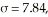find the 95% confidence interval for the population mean.

100)

____________

MULTIPLE CHOICE.  Choose the one alternative that best completes the statement or answers the question.

101) Suppose that you want to construct a 95% confidence interval for estimating a population mean.  How does the margin of error with a sample size of 100 compare with the margin of error with a sample size of 2500, if both samples have the same standard deviation?

101) _____

A) The margin of error for the first interval will be 5 times larger than the margin of error for the second interval.

B) The margin of error for the first interval will be 25 times larger than the margin of error for the second interval.

C) The margin of error for the second interval will be 25 times larger than the margin of error for the first interval.

D) The margin of error for the second interval will be 5 times larger than the margin of error for the first interval.

SHORT ANSWER.  Write the word or phrase that best completes each statement or answers the question.

102) A sociologist wishes to construct a confidence interval for the mean time spent on a social-networking website each week by  students at one college.  She selects a random sample of students of size n = 200. How would the width of a 90% confidence interval compare with the width of a 99% interval if both intervals are based on this same sample?

102)

____________

103) Which of the following statements about the t distribution is true?

103)

____________

104) Which of the following statements regarding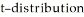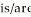true?

I.The total area under a t-distribution with 10 degrees of freedom is greater than the area under the

standard normal curve.

II.The t-distribution with 10 degrees of freedom is flatter and wider than the standard normal curve.

III.The t-distribution with 10 degrees of freedom more closely resembles the standard normal curve

than the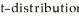with 20 degrees of freedom.

104)

____________

105) Suppose that you wish to obtain a confidence interval for a population mean. Under the conditions described below, should you use the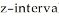, the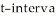, or neither?

? The population standard deviation is unknown.

? The population is normally distributed.

? The sample size is small.

105)

____________

## Solution 5 (1 Ratings )

Solved
Statistics 1 Year Ago 57 Views